# Gate Mock Test- 11: CS/IT

## 65 Questions MCQ Test GATE Computer Science Engineering(CSE) 2023 Mock Test Series | Gate Mock Test- 11: CS/IT

Description
Attempt Gate Mock Test- 11: CS/IT | 65 questions in 180 minutes | Mock test for GATE preparation | Free important questions MCQ to study GATE Computer Science Engineering(CSE) 2023 Mock Test Series for GATE Exam | Download free PDF with solutions
QUESTION: 1

### If the product of two integers 53 and 22 in a specific number system is 1276, then what is the decimal equivalent of the number 4371 in the same number system?

Solution: Since ‘6’ and ‘7’ have been used in the product of 53 and 22, the numbers have to be using base ‘8’ or ‘9’.

If we try with base ‘8’,-(53)8-= 8 × 5 + 3 = (43)10

-(22)8-=8 × 2 + 2 = (18)10

-43 × 18-= (774)10

whereas,-(1276)8 -=512×1+64 × 2+8 × 7+6 =(702)10

If we try with base ‘9’,-(53)9- = 9 × 5 + 3 =(48)10

-(22)9 - = 9 × 2 + 2 =(20)10

-48 × 20 -= (960)10

whereas,- (1276)9 - = 729 × 1 + 81 × 2 + 9 × 7+ 6 = (960)10

Converting-(4371)9 –

= 4 × (729) + 3 × (81) + 7 × (9) + 1 =3223.

QUESTION: 2

### If a and b are rational numbers and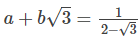, then a : b is

Solution:After rationalization,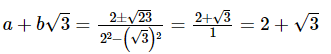On comparison, we get

a = 2

b = 1

a:b = 2:1

QUESTION: 3

### If the world ‘MAJORITY’ is encoded as ‘PKBNXSHQ’, how will the word ‘DAUGHTER’ be encoded?

Solution:

Divide the given word in 2 equal parts

M A J O R I T Y and each part is rewritten in reverse sequence

O J A M Y T I R.

Position of letters in the alphabet is 15/10/1/13 and 25/20/9/18 and position of letters in the given code PKBN/XSHQ is 16/11/2/14 and 24/19/8/17.

In comparison, you notice, the first half is obtained by adding ‘1’ and the second half is obtained by subtraction ‘1’.

QUESTION: 4

Which device is generally used in applications like ATMs, hospitals, airline reservation etc.?

Solution:
• A touch screen is a display that can recognize a touch to its surface area, either with a finger or a stylus.

• Touch screens are generally used in applications like ATMs, hospitals, airline reservations, supermarkets, etc.

QUESTION: 5

Direction: In these questions, the relationship between different elements is shown in the statements. These statements are followed by two conclusions.

Statement: A > B, B ≥ C = D < E

Conclusions:

I. C < A

II. D ≤ B

Solution: A > B, B ≥ C = D < E: A > B, B ≥ C → A>C & D= C, B ≥ C → D ≤B

Conclusion:

I. C > A: True

II. D ≤ B: True

QUESTION: 6

Direction: Study the following pie chart carefully to answer the question that follow:

Percentage of students enrolled in different streams in a college.

Total number of student = 3500Percentage break-up of girls enrolled in these stream out of the total students

Total number of girls = 1500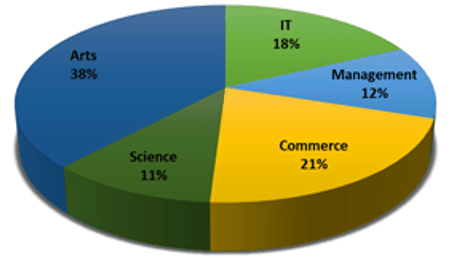What is the total number of boys enrolled in Management and IT together?

Solution:

The total number of boys enrolled in Management and IT together

= 3500 × 20 + 16100 - 1500 × 18 + 12100

= 35 × 36 - 15 × 30

= 1260 - 450 = 810

QUESTION: 7

In the following question, select the odd letters from the given alternatives.

Solution:

If we arrange alphabets in two rows as shown below, the letters are related as follows,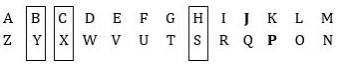Thus JP are the odd letters.

QUESTION: 8

Who was the first Viceroy of Portuguese in India?

Solution:
• Almeida was the first Viceroy of Portuguese in India .

•

Almeida took the island of Diu in his possession in AD 1509.

•

Albuquerque was the next Viceroy who won Goa in AD 1510.

•

Later on, the Portuguese also took Bassein & Daman in their possession.

QUESTION: 9

Direction: In the following question, the sentence is given with a blank to be filled in with an appropriate word. Select the correct alternative out of the four and indicate it by selecting the appropriate option.

Moving to the city was eye-__________ for the straitlaced country girl.

Solution:

Clearing means an open space in a forest, especially one cleared for cultivation.

Freeing means release from confinement or slavery.

Saving means release from confinement or slavery.

Eye-opening is a phrase meaning something that surprises you and teaches you new facts about life, people, etc.

QUESTION: 10

Select the option in which the given figure is embedded.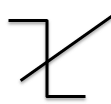Solution:

After carefully observing the figures given in the question, it is very clear that the question figure is embedded in answer figure (A). It is shown as given below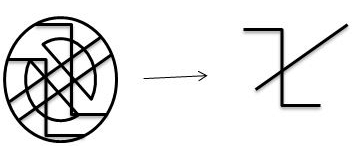Hence, Option A is the correct response.

QUESTION: 11

Serial communications involves interfacing of data among:

Solution: It exhibits serial exchange between microprocessor and peripherals such as printers, external drives, scanners or mice. The interface has parallel-to-serial converter which serves as data transmitter and serial-to-parallel converter which serves as data receiver.
QUESTION: 12

What is the wrong statement?

1) Complement of DFA that accepts those strings where after each ‘ a, always ‘ b ‘ appears, is the DFA that accepts those strings where after ‘ a ‘ never ‘ b ‘ appears.

2) In a language – no. of state in minimal NFA is n, then in worst case, maximum no. of state in equivalent DFA is 2n.

3) Minimal DFA always contains only one dead state.

Solution: For strings where after each ‘ a , always ‘ b ‘ appears, language is-

L1= {ab,abab,abb,bbab,.........,bbb,bbbbbb........}

For string where after ‘ a ‘ never ‘ b ‘ appears ,language is-

L2={ε,a,b,aa,ba,,bbb,bbbb.}

Some strings are there on intersection of L1 & L2 (Like bbb, bbbb etc)

So L1 & L 2 are not complementary to each other .

In a language – no. of state in minimal NFA is n, then in worst case, maximum no. of state in equivalent DFA is 2n.

More than one dead state gets combined during minimization of DFA, so DFA contains only one dead state.

QUESTION: 13

The number of 4 line to-16 line decoders required to make an 8-line-to-256 line decoder is

Solution: 8-line to-256-line decoder using 4-line to-16-line decoders is shown below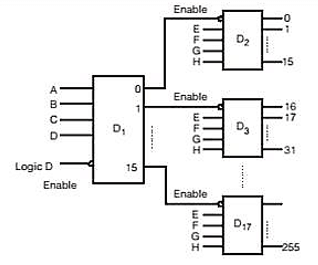Number of 4 x 16 MUX required

= 256 / 16 + 16/16 = 16 + 1 = 17

QUESTION: 14

Which of the following is a hardware generated signal.

Solution: A trap is a software-generated interrupt. An interrupt can be used to signal the completion of an I/O to obviate the need for device polling. A trap can be used to call operating system routines or to catch arithmetic errors.
QUESTION: 15

In an array of 2N elements that is both 2-ordered and 3-ordered, what is the maximum number of positions that an element can be from its position if the Arrays were 1-ordered?

Solution: An Arrays A [ 1 … n] is said to be k-ordered if

A [i - k] < A [ i ] < A [i + k]

for each i such that k < i < n – k.

For example, the Arrays 1 4 2 6 3 7 5 8 are 2- ordered.

Now come to question: Arrays are both 2 ordered and 3 ordered. i.e.

1 2 3 4 5 6 7 8

Now if the Arrays were 1-ordered then Arrays will be 1 2 3 4 5 6 7 8 maximum number of positions that an element can be from its position=1.

QUESTION: 16

Consider the following augmented grammar

S→aAb |eb

A→e |f

Find the number of states in LR(0) construction.

Solution: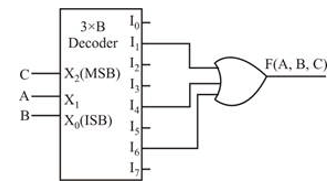No conflict in above DFA.

QUESTION: 17

Consider the following synchronous counter made up of JK, D and T flip-flops.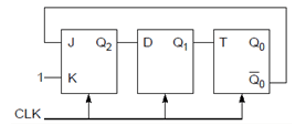Find the modulus value of the counter.

Solution: Consider characteristics equation of J-K flip-flop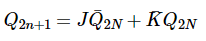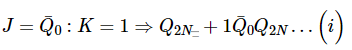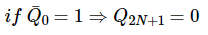Consider characteristic equation of D-Flip-Flop : QN+1=D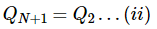Consider characteristics equation of T-Flip-flop: Q_N+1=T⊕Q_N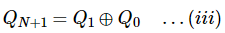Using equations (i), (ii) and (iii)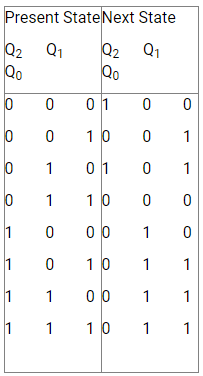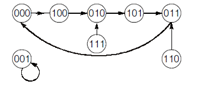The number of used states = 5

∴ Modulus value of the counter = 5.

QUESTION: 18

The maximum value of “a” such that the matrix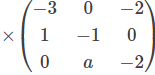has three linearly independent real eigenvectors is

Solution:

The characteristic equation of A is

|A-XI| = 0

⇒ f(x) = x3 + 6x2 + 11x + 6 + 2a

= (x + 1)(x + 2)(x + 3)+2a = 0

f(x) cannot have all 3 real roots (if any) equal

for if f(x) = (x-k)3, then comparing coefficients, we get

6 = –3k, 3k2 = 11

No such k exists

A) Thus f(x) = 0 has repeated (2) roots α, α, β

or

B) f(x) = 0 has real roots (distance) α, β, δ

Now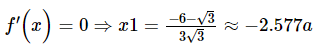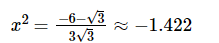At x1, f(x) has relative maximum

At x2, f(x) has relative minimum

The graph of f(x) will be as below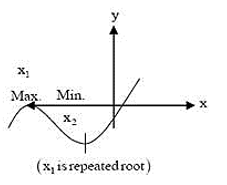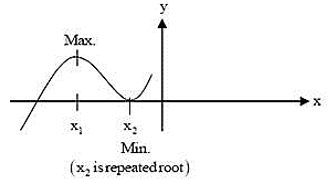Case A. repeated roots (α, α, β)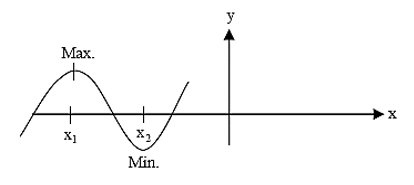Case B. distinct roots

Note that the graph of f(x) cannot be like the one given below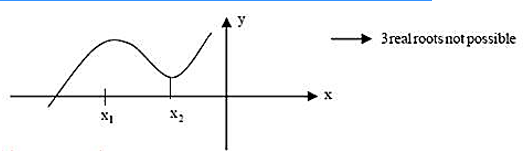Thus in all possible cares we have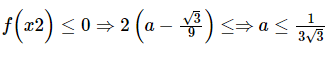QUESTION: 19

Match the following -

LIST- I

a. Token

b. Pattern

c. Lexeme

LIST – II

I) A rule describing the set of lexemes that can represent a particular token in the source program

II) A sequence of characters in the source program that is matched by the pattern for a token

III) A set of characters grouped together

Solution: Token is a set of characters grouped together like literal, id, num, const, if etc.

Pattern is a rule describing the set of lexemes that can represent a particular token in the source program. For example, if the token is related then its informal description of the pattern will be < or <= or = or > or >= or <> etc.

Lexeme is a sequence of characters in the source program that is matched by the pattern for a token. For example let the token be an identifier then its sample lexemes can be pi, count, a, b etc.

QUESTION: 20

The Servlet Response interface enables a servlet to formulate a response for a client using the method ___________.

Solution: void set Context type (String type)

- It’s used to send the content type of a response to be sent to a client, if the response has not been sent yet. The content type may include character encoding specifications.

QUESTION: 21

Let S = {1, 2, 3, . . . n} and G be a simple graph where every subset of S is a vertex in G. There will be an edge between two sub-set of S when they intersect in exactly 3 elements. What will be the degree of a vertex containing 4 elements?

Solution: Let the vertex having 4 elements is {1, 2, 3, 4}. Now select any 3 elements from this, say X = {1, 2, 3 ……..}, the number of ways to do this is 4C3.

Now for remaining n-4 vertices there is a choice whether to be included or not. So using the product rule, we get 2 × 2 × 2 × ….. (n-4) times.

So, we got the degree of the vertex having 4 elements is 4C3 x 2n-4

QUESTION: 22

The Breadth First Search algorithm has been implemented using the queue data structure. One possible order of visiting the nodes of the following graph if Q is starting node.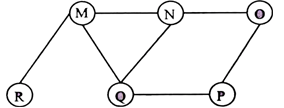Solution: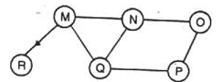Q →M

Q →N

Q →P

M→R

P →O

Using BFS Algorithm Order of visiting the nodes QMNPRO or QMNPOR

A) MNOPQR → If you try to run BFS, after M, you must traverse NQR (In some order) Here P is traversed before Q, which is wrong.

B) NQMPOR → This is also not BFS. P is traversed before O!

D) QMNPOR → Incorrect. Because R needs to be traversed before O.(Because M is ahead of N in the queue.

QUESTION: 23

A decimal number has 18 digits, The number of bits needed for its equivalent binary representation is(Approximately)?

Solution: Maximum number using 18 digits = 1018-1

1018-1= 2n

Take log both side

log10(1018-1) = log10(2n)

~ log10(1018) = log10(2n)

18 = n log102

n = 18/ log102

n = 59.79

~ n = 60

QUESTION: 24

Which one of the following is NOT a valid identity?

Solution:

(a) x ⊕ y = (xy + x′y ′)′

= (xy )′

= x ⊕ y, it is valid.

(b)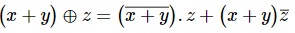=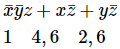= Σm(1, 2, 4, 6)

x⊕(y+z)=x ̅(y+z)+x((y+z) ̅ )

=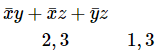= Σm(1, 2, 3, 4)

(x + y) ⊕ z ≠ x ⊕ (y + z)

So option (b) is invalid.

QUESTION: 25

Consider the following functions:

f(n)=2n

g(n)=n!

h(n)=nlogn

Which of the following statements about the asymptotic behaviour of f(n), g(n), and h(n) is true?

Solution:

For asymptotic behaviour

h(n) = O(f(n))

g(n) = Ω(f(n))

A function having its upper bound denoted by O notation

Ω notation provides a lower bound of asymptote.

QUESTION: 26

Husband and wife apply for two vacancies that arise in a private company. Two friends apply for that position. If the probability of 1st friend's selection and 2nd friend's selection are 3/5 and 1/6 respectively,

Find the probability of both friends getting rejected.

Solution:

Probability of 1st friend's selection is 3/5

Probability of 1st friend's rejection is (1-3/5)=2/5

Probability of 2nd friend's selection is 1/6

Probability of 2nd friend's rejection is 1-1/6=5/6

probability of both friends get rejected is (2/5)×(5/6)=1/3

QUESTION: 27

If A is 3 × 3 matrix, whose elements are given by aij = i2 – j2 where 1 ≤ i, j ≤ 3 then A–1 = _____

Solution:

aij = i2 – j2∀ i, j

∴ A3×3 is singular

∴ |A| = 0.

QUESTION: 28

Consider the system which have 3 processor p1, p2, p3 the pack demand for each 5, 9,13 for the resource 'R' then what is the minimum no of resources require to insure deadlock free operation

Solution:

P1 → 5 → 4

P2 → 9 → 8

P3 → 13 → 12

So P1 + P2 + P3 + 1 = 25.

QUESTION: 29

Consider the languages L1={wwR∣w∈{0,1}∗}

L2={w#wR∣w∈{0,1}∗}, where # is a special symbol

L3={ww∣w∈(0,1}∗)

Which one of the following is TRUE?

Solution: In formal language theory, a context-free language (CFL) is a language generated by some context-free grammar (CFG). Different CF grammars can generate the same CF language. It is important to distinguish properties of the language (intrinsic properties) from properties of a particular grammar (extrinsic properties). The set of all context-free languages is identical to the set of languages accepted by pushdown automata, which makes these languages amenable to parsing. Indeed, given a CFG, there is a direct way to produce a pushdown automaton for the grammar (and corresponding language), though going the other way (producing a grammar given an automaton) is not as direct.
QUESTION: 30

Consider the following code with 2-processes.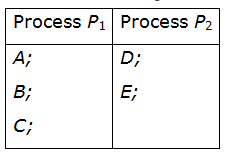If two processes are executing concurrently, which of the following execution orders never arise by them?

Solution: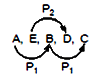order never arises.

Process P2 can execute D first then E. So option D. is correct.

QUESTION: 31

Mala has a colouring book in which each English letter is drawn two times. She wants to paint each of these 52 prints with one of k colours, such that the colour-pairs used to colour any two letters are different. Both prints of a letter can also be coloured with the same colour. What is the minimum value of k that satisfies this requirement?

Solution: This question is slightly ambiguous. So first let us understand what the question is asking. So in a book, we have letters A-Z and each letter is printed twice, so there are 52 letters. Now we have to colour each letter, so we need a pair of colours for that, because each letter is printed twice. Also in a pair, both colours can be some. Now the condition is that a pair of colours can’t be used more than once.

So suppose Mala has 3 colors : Red, Blue, Green. She can color as follows : (A,A) : (Red,Red), (B,B) : (Blue,Blue), (C,C) : (Green,Green), (D,D) : (Red,Blue), (E,E) : (Red,Green), (F,F) : (Blue,Green).

Now we don’t have more pairs of colours left, we have used all pairs, but could colour only 6 letters out of 26. So the question is to find the minimum no. of colours, so that we could color all 26 letters.

So if Mala has k colours, she can have k pairs of the same colours, thus colouring k letters, then kC2 other pairs of colours, thus colouring kC2 more letters.

So total no. of letters colored = k+kC2=k+k(k−1)2=k(k+1)2.

So we want k(k+1)2≥26 i.e. k(k+1)≥52, so k≥7, so 7 is the answer.

QUESTION: 32

Which of the following are context free? A=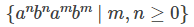B=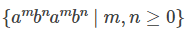C =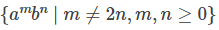Solution:
• A is context free : push a′s into stack, for each b's pop the stack. If stack is empty, then string is accepted

• B is NOT context free. Single stack is not sufficient because m and n are not comparable we cannot find when to push or pop;

• But this is CSL.

• C is context free : push a′s into stack, for each b's pop the stack two times. If the stack is empty, then the string is NOT accepted.

QUESTION: 33

Let L= {|M accepts some string} where M is a Turing machine. Find the language L?

Solution: Simulate M on all strings of length at most n for n steps and keep increasing n. We accept if the computation of M accepts some string. Language generated by a grammar is recursively enumerable hence it is turing recognizable. So it is also a recursively enumerable language.
QUESTION: 34

Which of the following relations is undecidable?

Solution: All problem in P are in NO(P⊂NP)

Whether P=NP or not undecidable in polynomial time. Reason is that every NP- problem does not have a solution in polynomial time. If any one proves that every NP- problem has a solution in polynomial time then P=NP otherwise by disproving it we get P!=NP.

: Option B is correct.

QUESTION: 35

Let A, B, C and D are problems. Consider the following polynomial reductions to know about the problem B.

(i) AB (A is reducible to B)

(ii) C D

(iii) B D

Find the correct statement from the following.

Solution:

A ≤ B

C ≤ D

B ≤ D

A. A is decidable ⇒ B need not be decidable.

B. D is undecidable ⇒ B need not be undecidable.

C. C is decidable ⇒ D need not be decidable.

D. A is undecidable ⇒ B is undecidable.

QUESTION: 36

Let G be a graph with 10 vertices, and d(v) be the degree of a vertex v. The following conditions are holds for graph G

(i) 3 ≤ d(v) ≤5 for each vertex v in G

(ii) Not every vertex degree is even

(iii) No two odd degree vertices are of the same degree.

How many vertices are even degree in G?

Solution:

8 vertices are having degree 4.

1 vertex with degree 3.

1 vertex with degree 5.

QUESTION: 37

Which of the following cases is definite to give O(nlog⁡n) time complexity always for quick sort to sort the array

A[0…n], for any set of values of array A Case 1: Choosing middle element as pivot. Case 2: Choosing pivot element as (2)th element initially followed by (21)th element, followed by (22)th element of array A and so on. Case 3: Choosing median element as pivot. Case 4: Choosing the pivot element randomly from the array A. Case 5: Choosing pivot such that the array is partitioned into almost two equal subarrays.

Solution:

Only case 3 and 5 ensures O(nlog⁡n) time complexity always.

Case 1: Choosing middle element doesn't mean that pivots location is in middle, after the

partition algorithm. In the worst case pivot may go either first or last location (O(n2)).

Similarly Case 2, Case 4 does not guarantee O(nlog⁡n). But cases 3 and 5 are similar and make sure that pivot always goes to the middle location, after the partition algorithm.

QUESTION: 38

The following code is a naïve implementation of the LCS problem. What is the complexity of the algorithm if tabulation is implemented?

/* Returns length of LCS for X[0..m-1], Y[0..n-1] */

int lcs( char *X, char *Y, int m, int n )

{

if (m == 0 || n == 0)

return 0;

if (X[m-1] == Y[n-1])

return 1 + lcs(X, Y, m-1, n-1);

else

return max(lcs(X, Y, m, n-1), lcs(X, Y, m-1, n));

}

Solution:

int lcs( char *X, char *Y, int m, int n )

{

int L[m+1][n+1];

int i, j;

/* Following steps build L[m+1][n+1] in bottom up fashion. Note

that L[i][j] contains length of LCS of X[0..i-1] and Y[0..j-1] */

for (i=0; i<=m; i++)

{

for (j=0; j<=n; j++)

{

if (i == 0 || j == 0)

L[i][j] = 0;

else if (X[i-1] == Y[j-1])

L[i][j] = L[i-1][j-1] + 1;

else

L[i][j] = max(L[i-1][j], L[i][j-1]);

}

}

/* L[m][n] contains length of LCS for X[0..n-1] and Y[0..m-1] */

return L[m][n];

}

Hence when tabulation is used , distinct function calls = (m+1)(n+1)

Hence time complexity = O(mn).

QUESTION: 39

Consider the following SDT.

C→Co S {C. val =C1. Val+ S.val}

C→S {C. val = S. val}

S→So E { S. val = S1.Val×E.val}

S→E { S. val + E. val}

E→id { E.val = idnum}

Find the value of input expression

“2o3o5o3o1o3”.

Solution: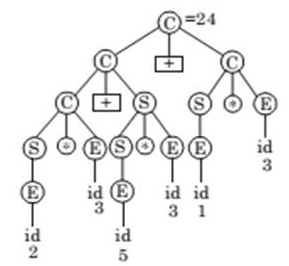QUESTION: 40

In the C program given below, find the number of tokens present in it.

int main()

{

int l = 5, m= 4;

printf(“abbc ok : %d”, l*m);

return 0;

}

Solution:

Lexical analyzer 1st reads int and finds it valid as a token then proceeds further

So ‘int’,’ main’,’(‘,’)’,’{‘,’int’,’l’,’=’,’5’,’m’,’=’,’4’ then counting the further tokens in the similar way leads to a total count of 32, Hence option b is correct.

QUESTION: 41

Given a set of functional dependencies on R (A, B, C, D, E) find the number of candidate keys?

A ⇒ B

BC ⇒ E

DE ⇒ A

Solution:

Candidate keys - ACD, BCD, ECD

QUESTION: 42

Consider the below given C program :

#include

#include

enum {false, true};

int main()

{

int i = 1;

do

{

printf("%d\n", i);

i++;

if (i < 15)

continue;

} while (false);

getchar();

return 0;

}

How many times the print statement is executed _________.

Solution:

The do while loop checks condition after each iteration. So after the continue statement, control transfers to the statement while(false). Since the condition is false ‘i’ is printed only once.

QUESTION: 43

What does the following code do if the head of a singly linked list is passed as a parameter:

void ABC(struct Node **head_ref, int position)

{

return;

if (position == 0)

{

free(temp);

return;

}

for (int i=0; temp!=NULL && i

temp = temp->next;

if (temp == NULL || temp->next == NULL)

return;

struct Node *next = temp->next->next;

free(temp->next);

temp->next = next;

}

Solution:

The code deletes the element at the given position.

We can verify this by taking a specific value of position.

QUESTION: 44

Consider the following statements about Bipartite graphs.

(a) The maximum number of edges in a bipartite graph with 10 vertices is

(b) The maximum number of edges in a bipartite graph with 24 vertices is 143.

(c) The minimum number of edges in a complete bipartite graph with 10 vertices is 9.

(d) Every bipartite graph is 2-colorable.

The number of correct statements is _______________?

Solution:

In a bipartite graph, the maximum edges will be present when both the partitions have equal number of vertices.

That’s means with 10 vertices the maximum no. of edges we can have is 25(5*5).

With 24 vertices the maximum no. of edges we can have is 144. That is 12 * 12.

Both the first and 2nd statements are wrong.

Statement 3 is true, because we have minimum edges in a complete graph when one partition has only 1 vertex and the other partition has 9 vertices. So the number of edges is 9.

Statement 4 is true, Because color the one partition with one color and other partition with other color. As there is no edge between the elements of a single partition, there will be no problem.

QUESTION: 45

A group (G, *) is called a cyclic group if there exists an element a∈G, such that every element of ‘G’ can be written an for some integer n. Then ‘a’ is called a generating element / generator. Consider the following group G = ( {1, 2, 3, 4, 5, 6},⊗7), , where ⊗7is multiplication modulo 7 operation. Let X be the number of generators of G. And Y be the sum of these generators. Find X + Y is _________________?

Solution:

G = ( {1, 2, 3, 4, 5, 6}, ⊗7)

The number of generators of a group (if there exist any) is Sn. Where Sn is the set of all +ve integers which are less than n and relatively prime to n. Also, n is the number of elements in G.

S6 = {1, 5} So 2 generators are there for G.

X = 2.

Y = 3 + 5 = 8, because 3 and 5 are the generators of G.

X + Y = 10.

QUESTION: 46

Consider the following groups

(1) G1 = ( {1, 3, 5, 7}, ⊗8) , where ⊗8 is multiplication modulo 8 operation.

(2) G2 = ( {1, 2, 3, 4, 5, 6}, ⊗7), where ⊗7is multiplication modulo 7 operation.

(3) G3 = ( {0, 1, 2, 3, 4},⊗5)where ⊗5) is addition modulo 5 operation.

(4) G4 = ( {1, 2, 4, 5, 7, 8}, ⊗9), where ⊗9 is multiplication modulo 9 operation.

The number of Cyclic groups is ___________________?

Solution:

A group (G, *) is called a cyclic group if there exists an element a∈G, such that every element of ‘G’ can be written an for some integer n. Then ‘a’ is called a generating element / generator.

G1 do not have any generators. So, it is not a cyclic group.

G2 have 3 and 5 as generators.

G3 have 1, 2, 3 and 4 as generators.

G4 also has a generator. One generator is enough to say whether a group is cyclic or not. Here 2 is the generator.

QUESTION: 47

Suppose you went on a trip with your friends in a car. There are five seats in a car. You and your friends total count to 5 also. Each of you knows how to drive. You all stop the car at a restaurant. After coming back from the restaurant, you all decided not to sit on the same seat instead of sitting on each other's seats. No one can sit in the same seats they were sitting before the stoppage.

In how many ways can this be done _____________________?

Solution:

For ex: The permutation 12345 is a derangement of 54231.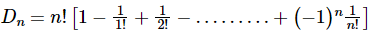D5= 44.

QUESTION: 48

Consider transaction T0 and T1 which are defined as:

A = A – 50;

write(A);

B : = B + 50;

Write(B);

C: = C – 100;

Write(C);

A, B, C before execution were Rs. 1000, Rs. 2000, Rs. 700 respectively and the system crash occurs after write (B), then which of the following is correct?

A = 950

B = 2050

Solution:

The log at the time of the crash appears as in option (c). When the system comes back up, no redo action needs to be taken, since no commit record appears in the log. The values of A and B remain Rs. 1000 and Rs. 2000 respectively.

QUESTION: 49

What are the outputs of the following program?

main()

{

int u =1;

int v = 3;

printf(“u = %d”, v =%d”, u, v);

funct1(u, v);

printf(“u = %d”, v = %d”, u, v);

funct2(&u, &v);

printf(“u = %d”, v = %d”, u, v);

}

funct1( int u, int v)

{

u = u + v;

v = u – v;

u = u – v;

}

funct2( int *pu, int *pv)

{ *pu = *pu + *pv;

*pv = *pu - *pv;

*pu = *pu - *pv;

}

Solution:

First printf prints u = 1 v = 3

Again funct1 (u, v) in called

→ funct1(1, 3)

→ u = u + v = 1 + 3 = 4

v = u – v = 4 -3 = 1

u = u – v = 4 – 1 = 3

Again printf will print u = 1 v = 3 because values of u and v actually have not changed

When funct2 (&u, &v); is called

And it is called reference hence the values of u and v are actually interchanged

→ When third printf (c) is executed, the values of u and v are actually interchanged

QUESTION: 50

Consider a reduced 7-bit IEEE floating-point format, with 3 bits for the exponent and 3 bits for the significand (Mantissa).What will be the value which get stored in the system corresponding to (-0.5625)?

Solution:

The format can be specified as follows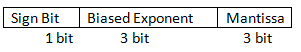Here, Sign Bit=1

Also, 0.5625= (0.1001)

For normalised form,

0.5625= 1.001 * 2-1

Hence Actual Exponent= -1

Biased Exponent= Actual Exponent + Bias

Bias= largest signed integer possible with 3 bits

= 3

Biased Exponent= 3-1

= 2 (010)

Therefore, Binary representation will be (1010001).

QUESTION: 51

Consider the following function, to which a pointer to the root node of the binary tree T is passed.

int Gradeup (Struct Node * root)

{

int a = 0, b = 0, c = 0;

if(root == NULL) return 0;

if(root → left == NULL && root → right == NULL) return 1;

a = Gradeup (root → left);

b = Gradeup (root → right);

c = 1 + max(a, b);

return c;

}

The above program computes,

Solution:

The above program computes the number of levels in T, Option (B) is actually the definition of the number of levels (or depth) of a tree. For example, if the tree T has only one node, it returns 1. So options (A) and (C) are eliminated, as if (A) was supposed to be true, it should have returned 0. Now the only dilemma is between (B) and (D), which can also be handled easily, as the line C = 1 + max (a, B. should have been c = 1 + a + b instead, but that's not the case and therefore it only computes the number of levels in T. All this explanation is given to help you solve the question in the exam quickly, but with a lot of practice, just by looking at the code you will be able to tell what the function can do and cannot do.

QUESTION: 52

A Finite State Machine can be used to add how many given integers?

Solution:

A Finite State Machine can be designed to add two integers of any arbitrary length (arbitrary number of digits).

Hence, the correct answer is 2.

QUESTION: 53

The speed gained by an ‘n’ segment pipeline executing ‘m’ tasks is

Solution:

Stages in pipeline = n

Without pipelining the number of cycles required to execute m tasks = nm.

(∵ each task required n cycle)

When we pipeline the tasks for Ist task it requires n cycles and for next (m - 1) cycle for each (m - 1) tasks.

So total cycles required with pipelining = n + (m – 1) × 1

= (n + m – 1)

∴ Speed gained by pipeline =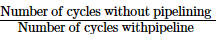=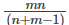QUESTION: 54

A computer uses polynomials for error detecting and uses generator G(x) as the generator polynomials to generate the check bits. G(x) and message M(x) that are to be transmitted is given below:

G(x) : X4 + X3 + 1

M(x) : x6 + x4 + x2 + x

Which of the following remainder bits will be added while transmitting the message M(x) ?

Solution:

Given,

G(x) : X4 + X3 + 1 = 11001

M(x) : x6 + x4 + x2 + x

= 1010110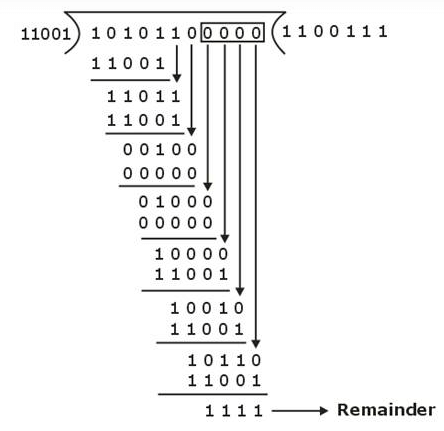Transmitted frame = 10101101111

QUESTION: 55

Consider the array A = < 4, 1, 3, 2, 16, 9, 10, 14, 8, 7 >. After building a max heap from the array A, the height (the longest path from root to the leaf) of the heap and the sum of elements present in the root’s right subtree are equal to _______ and _______. (Root is at level 0)

Solution:

We can apply build max heap and the resultant max heap will have height equal to 3, and the elements present in the right subtree will be 10, 3, 9.

Therefore the sum will be equal to 10 + 3 + 9 = 22.

QUESTION: 56

Let A be matrix such that,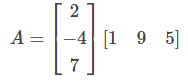Let x, y and z be the eigen value of A. Then the product xyz is equal to ________.

Solution:

Product of eigenvalues of a matrix A = Determinant of A

Upon evaluation, we see that |A| = 0

⇒ x.y.z = |A| = 0

QUESTION: 57

What is the minimum level of B tree index required for storing 7500 key and order of B tree is (Order is maximum child pointer a node can have) ________.

Solution:

Order of B tree = 6

Maximum key in one node = 5

1st level – 5 key

2nd level – 6 × 5 key

3rd level – 6 × 6 × 5 key

4th level – 6 × 6 × 6 × 5 key

5th level – 6 × 6 × 6 × 6 × 5 key

Total keys in five level = 5 + 30 + 180 + 1080 + 6480

= 7775

Total 5 levels required.

QUESTION: 58

Consider the following set of processes that need to be scheduled on a single CPU operating system using the preemptive shortest remaining time first algorithm. (Tries are broken on first come first serve basis)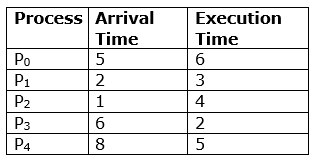(All time in milliseconds)

The average waiting time of these processes are ________ (in milliseconds). (Upto 1 decimal place)

Solution: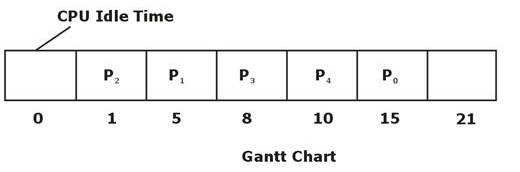Waiting Time = Turnaround Time - Execution (burst) time

Average waiting Time =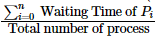=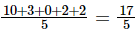= 3.4ms

QUESTION: 59

Consider the following statement given below:

S1: Inter process communication and sharing is easier between processes than threads.

S2: There is no protection between threads of the same process.

Which of the above statements are incorrect?

Solution:

S1 : Inter process communication and sharing is easier between threads than process, S1 incorrect

S2 : Threads share the same address space in process so there is no protection between threads. S2 correct

QUESTION: 60

Let G be a directed graph whose vertex set contains numbers from 1 to 1024. There is an edge from a vertex i to a vertex j iff either j = I + 1 or j = 3i. The minimum number of edges in the path from vertex 1 to vertex 100 is

Solution:

The shortest path between 1 and 100 is 1 → 3 → 9 → 10 → 11 → 33 → 99 → 100

And the number of edges in this path = 7

Hence the answer is option (d).

QUESTION: 61

Consider the following C function:

void foo(int n)

{

while (n! = 0)

{

if (!(n & 1))

printf(“*”)

n = n > > 1;

}

}

The number of times printf(“*”) statement is executed, when the value 224 is passed to the function foo( ) is ________.

Solution:

The above function, prints * as many times as the number of zeroes in the binary representation of n. In 224, the bit pattern is 1024 (1 followed by 24 zeros), and thus 24 stars are printed by the above function.

QUESTION: 62

Consider the following statements regarding alphabet and language inequalities.

S1:∑∗−{∈}=∑+

S2:L∗−{∈}=L+

Which of the above statements are always true?

Solution:

Only S1 holds true. The problem in S2 is that, if "" belongs to L, RHB will contain , but LHS won't, so the equality will not hold, Therefore S2 will be false.

QUESTION: 63

A hypothetical processor contains 25 registers and 110 opcodes. Each instruction of the processor has four fields namely opcode, 2 register operands and 1 for direct addressing. The number of bits is used to represent the direct addressing field when the 30 bit word size used is ________.

Solution:

Number of register = 25

= [log2 25] = 5

Number of opcodes = 110

= [log2 110] = 7

Instruction representation :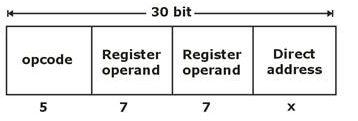30 = 5 + 7 + 7 + x

x = 11 bit

QUESTION: 64

Which of the given choices is the correct time complexity of the following code?

for (k = 1; k < (n + 1); k++)

for (m = 1; m < (n + 1); m + = k)

x = x + 1;

Solution:

\begin{tabular}{l|l|l|l|l}

k=1 & k=2 & k=3 & ……… & k=n \

times & n/2 times & n/3 times & & 1 times

\end{tabular}

Hence time complexity T(n)

=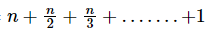=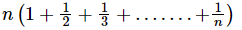= n(log n)

= θ(n log n)

QUESTION: 65

Consider the following regular expressions over the alphabet {0, 1}.

I. 1* 0(0 + 1)*

II. (0 + 1)* 01*

III. 1* 0(1 + 0)* + (0 + 1)* 01*

Which of the above regular expressions are equivalent?

Solution:

I and II are quite easy to understand - both denote strings containing at least one 0. III is actually the union of I and II, but III is again denoting strings containing at least one 0, as both regular expressions (i.e. I and II) are equivalent, so union won't make any difference.

Hence all 3 regular expressions are equivalent.Use Code STAYHOME200 and get INR 200 additional OFF Use Coupon Code